Courses

# Test: General Properties Of 17Th Group (Halogens)

## 25 Questions MCQ Test Chemistry Class 12 | Test: General Properties Of 17Th Group (Halogens)

Description
This mock test of Test: General Properties Of 17Th Group (Halogens) for JEE helps you for every JEE entrance exam. This contains 25 Multiple Choice Questions for JEE Test: General Properties Of 17Th Group (Halogens) (mcq) to study with solutions a complete question bank. The solved questions answers in this Test: General Properties Of 17Th Group (Halogens) quiz give you a good mix of easy questions and tough questions. JEE students definitely take this Test: General Properties Of 17Th Group (Halogens) exercise for a better result in the exam. You can find other Test: General Properties Of 17Th Group (Halogens) extra questions, long questions & short questions for JEE on EduRev as well by searching above.
QUESTION: 1

### Direction (Q. Nos. 1-10) This section contains 10 multiple choice questions. Each question has four choices (a), (b), (c) and (d), out of which ONLY ONE is correct. Q. Which of the following property decreases down the group in the halogens?

Solution:

Due to increase in atomic size, ionisation enthalpy decreases down the group.

QUESTION: 2

### Which of the following represents the decreasing order of van der Waals’ forces in halogens ?

Solution:

Melting point increases with halogens from fluorine to iodine because van der Waals’ forces are stronger down the groups.

QUESTION: 3

### The correct order of in creasing order of radiiions Br- , F-, O2- and S2-  is as follow

Solution:

Atomic and ionic radii increase down the group due to increasing number of quantum shells.

QUESTION: 4

The correct order for the percentage of ionic character is

Solution:

As the electronegativity of halogen decreases, the dipole moment of the hydrogen halide and hence, the ionic character decreases in the same order, i.e. HI < HBr < HCI < HF.

QUESTION: 5

Which of the following statement is incorrect ?

Solution:

The correct order of bond dissociation enthalpy is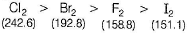QUESTION: 6

Which option is incorrect among the following for the given property?

Solution:

Thermal stability decreases as the strength of H-X bond decreases which in turn decreases as the size of the halogen increases, i.e. HF > HCI > HBr > HI

QUESTION: 7

When Cl2 gas reacts with hot and concentrated sodium hydroxide solution, the oxidation number of chlorine changes from

Solution:

When chlorine gas reacts with hot and concentrate NaOH solution, it disproportionates into Chloride (Cl-) and Chlorate (ClO3-) ions.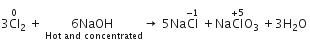QUESTION: 8

Which of the following does not exist?

Solution:

Fluorine has no d-orbitals in the valence shell. Hence, it always show - 1 oxidation state and monovalency in its compounds.

QUESTION: 9

One gas bleaches the colour of flowers by reduction and another gas by oxidation. The gases respectively are

Solution:

Cl2 bleaches by oxidation while SO2 does it by reduction.

QUESTION: 10

Solid iodine is an example of

Solution:

Solid iodine is a molecular solid.

*Multiple options can be correct
QUESTION: 11

Direction (Q. Nos. 11-15) This section contains 5 multiple choice questions. Each question has four choices (a), (b), (c) and (d), out of which ONE or MORE THAN ONE are correct.

Q.

The fluoride ores are

Solution:

The cryolite (Na3AIF6) and fluorspar (CaF2) are two ores of fluoride.

*Multiple options can be correct
QUESTION: 12

Which one of the following arrangements do not give the correct picture of the trends indicated against it?

Solution:

The negative electron gain enthalpy of fluorine is less than that of chlorine. It is due to small size of fluorine atom.

*Multiple options can be correct
QUESTION: 13

Which of the following are insoluble in water?

Solution:

The correct answers are option A,B and D.
The number of halides which are insoluble in water is 3.
They include AgCl, AgBr, AgI
Covalent compounds are insoluble in water. Therefore, AgF will be the most ionic compound and so it will be most soluble in water while AgI will be the least soluble.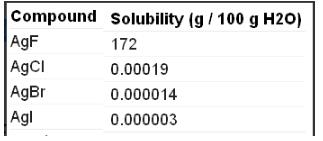*Multiple options can be correct
QUESTION: 14

The source of iodine is

Solution:

Explanation : Fish (such as cod and tuna), seaweed, shrimp, and other seafood, which are generally rich in iodine. Dairy products (such as milk, yogurt, and cheese) and products made from grains (like breads and cereals), which are the major sources of iodine in American diets.

Chile saltpeter (NaNO3) contains 5-20 % NaIO3. Chile saltpeter is also known as caliche. It is an important source of iodine.

*Multiple options can be correct
QUESTION: 15

When chlorine reacts with hot, cone. NaOH, the products formed are

Solution: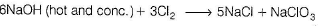+ 3H2O

QUESTION: 16

Direction (Q. Nos. 16 and 17) This section contains a paragraph, describing theory, experiments, data, etc. Two questions related to the paragraph have been given. Each question has only one correct answer among the four given options (a), (b), (c) and (d).

Passage

Group 17 elements are known as halogens ( sea salt forming). Their general configuration is ns2np5 where, n = 2 to 7. Fluorine is the first member of the group differs in several ways from the rest of the group. Halogens are highly reactive elements having strong affinity for hydrogen. All the halogens form both ionic and covalent compounds .Fluorine is always monovalent and shows -1 oxidation state in its compounds .The other halogens exhibit 1, 3, 5, 7 valencies in their compounds and show - 1 to + 7 oxidation states.

Q. Valence shell configuration that belongs to most reactive non-metal is

Solution:

All the halogens are highly reactive. They react with metals and non-metals to form halides due to readily acceptance of an electron

QUESTION: 17

Passage

Group 17 elements are known as halogens ( sea salt forming). Their general configuration is ns2np5 where, n = 2 to 7. Fluorine is the first member of the group differs in several ways from the rest of the group. Halogens are highly reactive elements having strong affinity for hydrogen. All the halogens form both ionic and covalent compounds .Fluorine is always monovalent and shows -1 oxidation state in its compounds .The other halogens exhibit 1, 3, 5, 7 valencies in their compounds and show - 1 to + 7 oxidation states.

Q.

In which of the following chlorine has different oxidation states?

Solution:

In calcium oxychloride (CaOCI)CI) has different oxidation states, Hence, chlorine has +1 and -1 oxidation states.

QUESTION: 18

Direction (Q. No. 18 and 19) Choices for the correct combination of elements from Column I and Column II are given as options (a), (b), (c) and (d), out of which one is correct.

Q.

Match the Column I with Column II and mark the correct option from the codes given below.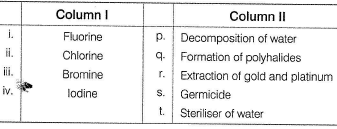Solution: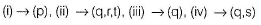QUESTION: 19

Match the Column I with Column II and mark the correct option from the codes given below.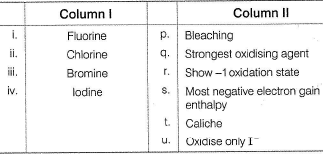Solution: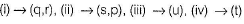*Answer can only contain numeric values
QUESTION: 20

Direction (Q, Nos. 20-24) This section contains 5 questions. When worked out will result in an integer from 0 to 9 (both inclusive).

Q.

The num ber of lone pair of electrons present on the central iodine in I-3 ion is

Solution:

Three lone pairs are present on the central iodine in triiodide ion.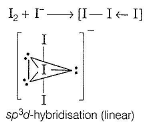*Answer can only contain numeric values
QUESTION: 21

In the MOT of F2 molecule, number of electrons occupying antibonding orbitals are

Solution:

Fluorine atom have 2+7 electrons so an F2 molecule contain 18 electrons.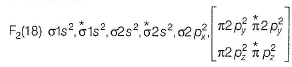Hence, 8 electrons occupy the antibonding orbitals.

*Answer can only contain numeric values
QUESTION: 22

The total number of positive oxidation states shown by fluorine is

Solution:

Fluorine show only one positive oxidation state of +1 in HOF while all of the halogens show positive oxidation state of +1, +3, +5 and +7.

*Answer can only contain numeric values
QUESTION: 23

When carnallite is dissolved in water the number of ions formed are

Solution:

Carnallite is a double salt. It will dissociate into simple substances or ions completely when dissolved in water.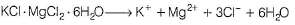Hence, 5 ions are produced.

*Answer can only contain numeric values
QUESTION: 24

The number of water insoluble salts among

AgF, AgCI, AgBr, Agl BeF2, CaF2,KF, PbCI2, HgCI2

Solution:

AgCI, AgBr, Agl CaF2, PbCI2, HgCI2, are water insoluble.

QUESTION: 25

Direction (Q. No. 25) This section is based on Statement I and Statement II. Select the correct answer from the codes given below.

Q.

Statement I : All halogens are coloured.

Statement II : Halogens absorbs part of the light in the visible region.

Solution:

Halogen molecule absorbs light in the visible region as a result of which their electrons are excited to higher energy level while the remaining light is transmitted.
The colour of halogens is actually the colour of this transmitted light, i.e. halogens have complementary colours.